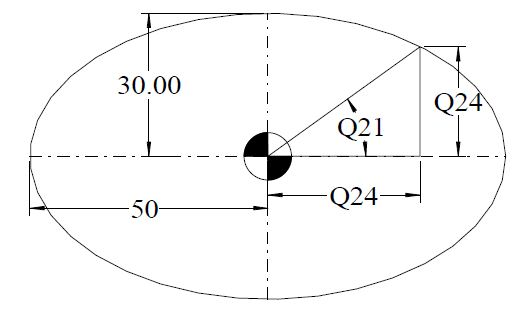# G65 Macro for Internal Elipse

Submit by: Machinist

## Drawing/Image## CNC Program

```T1 M6
G0 G90 G40 G21 G17 G94 G80
G54 X0 Y0 S? M3
G43 Z5 H?
G1 Z-? F?
#20 = 2 ; Incremental degree calculation
#21 = 0 ; Start Angle
#22 = 30 ; Y Axis Radius
#23 = 50 ; X Axis Radius
G41 X#23 D? ; Compensation motion to right side of internal pocket
N10 #21 = [#21 + #20] ; Angular Count
#24 = SIN[#21] ; Incremental Y axis calculation
#25 = COS[#21] ; Incremental X axis calculation
#24 = [#24*#22] ; Absolute Y calculation
#25 = [#25*#23] ; Absolute X calculation
X#25 Y#24 ; Movement in X & Y axis
IF [#21 LT 360] GOTO 10 ; Restart if less than 360 degree motion
IF [#21 GT 360] GOTO 20 ; If final angle becomes greater than 360 degrees recalculate
IF [#21 EQ 360] GOTO 30 ; Finish if total angle is equal to 360 degree
N20 #21 = 360
GOTO 10
N30 G40 X0
G0 G90 Z100 M30```